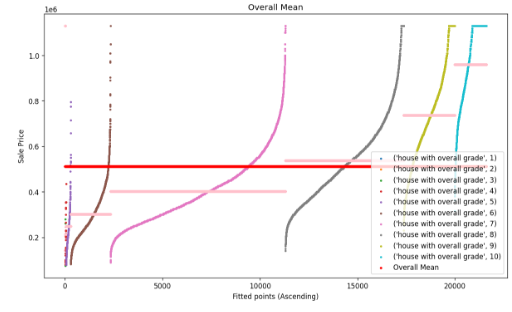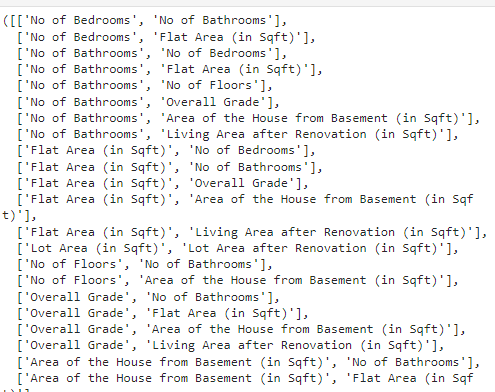top of page
• rishabhdwivedi062

# House price prediction using linear regression model

We will be building a House Price prediction model using linear regression, I will try to make everything simple for you.

You can access the dataset from here: Click me

Part 1: Building your first predictive model with a mean prediction

Import the necessary python libraries

```import pandas as pd
import numpy as np
import matplotlib.pyplot as plt
import seaborn as sns```

```data = pd.read_csv('Transformed_Housing_Data2.csv')
data.head() # printing the first 5 rows```We have multiple columns which are like No. of Bedrooms. , No. of Bathrooms, etc

Add a new column that contains the mean sale price.

```data['Mean_sales']= data['Sale_Price'].mean()
data['Mean_sales'].head() # print first 5 rows```The mean sale price is the same for every house, we need a bit of improvement here.

```# Plotting and visualising the data
plt.figure(dpi=100)
k=range(0,len(data))
plt.scatter(
k,data['Sale_Price'].sort_values(),
color='red',
label='Actual Sale Price')
plt.plot(
k,data['Mean_sales'].sort_values(),
color='green',
label='Mean Sale Price')
plt.xlabel('Fitted points (Ascending)')
plt.ylabel('Sale price')
plt.title('Overall Mean')
plt.legend()

# We are making graph for Actual sale price and mean sale price```Conclusion: As the mean price is constant, so we can use it as a good predictor for very high-priced and very low-priced houses.

Part 2: Improvement of the mean regression model.

We will use the concept of Grade mean now, it will group similar mean values

together and assign a new mean value.

```# For example
values='Sale_Price',
aggfunc=np.mean)

Applying the same concept on our dataset

```# Making new column
# for every grade fill its mean price in new columnLet's visualize the things

```# visualizing
for i in range(1,11):

```classwise_list = []
for i in range(1,11):
classwise_list.append(k)```

```plt.figure(dpi=120,figsize=(12,7))
# z variable is for x-axis

z=0
for i in range(1,11):
points=[k for k in range(z,z+len(classwise_list[i-1]))]

plt.scatter(points,
classwise_list[i-1].sort_values(),
,s=4)

plt.scatter(points,
[classwise_list[i-1].mean()
for q in range(len(classwise_list[i-1]))],
s=6,color='pink')

############ Plotting overall mean ##########
plt.scatter([q for q in range(0,z)],
data['Mean_sales'],
color='red',
label='Overall Mean',
s=6)

plt.xlabel("Fitted points (Ascending)")
plt.ylabel("Sale Price")
plt.title("Overall Mean")
plt.legend(loc=4)```This plot shows the most precise prices.

Part 3: Residual Plot.

We will be making a residual plot, which will be the difference between grade mean and sale price.

```mean_difference = data['Mean_sales']- data['Sale_Price']

```k = range(0,len(data))
l = [0 for i in range(len(data))]

plt.figure(figsize=(15,6),dpi=100)

plt.subplot(1,2,1)
plt.scatter(
k,
mean_difference,
color='red',
label='Residual',
s=2
)
plt.plot(k,l,color='green',label='Mean Regression',linewidth=3)
plt.xlabel("Fitted points")
plt.ylabel('Residuals')
plt.title("Residual with respect to grade wise mean")

plt.subplot(1,2,2)
plt.scatter(
k,
color='red',
label='Residual',
s=2
)
plt.plot(k,l,color='green',label='Mean Regression',linewidth=3)
plt.xlabel("Fitted points")
plt.ylabel('Residuals')
plt.title("Residual with respect to grade wise mean")```Conclusion: The first model has a high residual as it is more spread. Hence model 2 is the perfect model.

##### Model Evaluation Metrics

```# Calculating Mean Error
cost = sum(mean_difference)/len(data)
print(round(cost,7))```

Output : 0.0

To overcome this problem we will use another MEM.

Mean Absolute Error

```Y= data['Sale_Price']
Y_hat1= data['Mean_sales']
n= len(data)

from sklearn.metrics import mean_absolute_error

Output: 137081.7029820291

Mean Squared Error (MSE)

```from sklearn.metrics import mean_squared_error
cost_mean=mean_squared_error(Y_hat1,Y)

Output: (62528116847.799576, 30804835720.342426)

Let's fine-tune the model, treat multicollinearity and build the Linear regression model.

Scaling the dataset

```from sklearn.preprocessing import StandardScaler
scaler = StandardScaler()
Y= data['Sale_Price']  # target variable
X = scaler.fit_transform(data.drop(columns=['Sale_Price']))
X = pd.DataFrame(data=X,columns=data.drop(columns=['Sale_Price']).columns)```# Checking and removing Multicollinearity
X.corr()``````# pair of independent variables with corellation greater then 0.5
k = X.corr()
z= [[str(i),str(j)] for i in k.columns for j in k.columns if (k.loc[i,j]>0.5 and (i!=j))]
z,len(z)```Calculating VIF

```from statsmodels.stats.outliers_influence import variance_inflation_factor
vif_data=X

# calculating VIF for each column
VIF = pd.Series([variance_inflation_factor(vif_data.values,i) for i in range(vif_data.shape)],index=vif_data.columns)
VIF````VIF[VIF==VIF.max()].index`

Output: 'Flat Area (in Sqft)'

```# Let's make a function for it

def MC_remover(data):
vif = pd.Series([ variance_inflation_factor(data.values,i) for i in range(data.shape)],index=data.columns)
if vif.max()>5:
print(vif[vif==vif.max()].index,"has been removed")
data = data.drop(columns=[vif[vif==vif.max()].index])
return data
else:
print("No multicollinerty present anymore")
return data```

```for i in range(7):
vif_data = MC_remover(vif_data)

Output:

Flat Area (in Sqft) has been removed Condition_of_the_House_Fair has been removed No multicollinerty present anymore No multicollinerty present anymore No multicollinerty present anymore No multicollinerty present anymore No multicollinerty present anymore

```VIF = pd.Series([variance_inflation_factor(vif_data.values,i) for i in range(vif_data.shape)],
index=vif_data.columns)
VIF,len(VIF)```Train/Test Set

```X=vif_data
y=data['Sale_Price']```

```from sklearn.model_selection import train_test_split
X_train,X_test,y_train,y_test = train_test_split(X,y,test_size=0.3,random_state=101)
X_train.shape,X_test.shape,y_train.shape,y_test.shape```

Output: ((15126, 28), (6483, 28), (15126,), (6483,))

Linear Regression:

```from sklearn.linear_model import LinearRegression
lr = LinearRegression(normalize=True)
lr.fit(X_train,y_train)
# lr.fit()-->this function implements the gradient descent and the complete procedure over the training data```

```lr.coef_
# coefficient is nothing but y=m1x1+m2x2.....
# Since data is normalized therefore m0=0```

```Output
array([ -3928.66247639,  12028.44560689,  14967.00497585,   2697.55278605,
27220.31313417,  59965.44665815,  80697.80906997,  27729.56715434,
27873.90231343,  21397.40341959, -23854.32640243,  17943.26729788,
-2896.98542901, -10179.085198  ,  14239.3533334 ,   5095.97603572,
-2296.64888137,  14594.33847962,  10761.77007875,  12165.83372082,
33842.29544383,  63269.82875283,  81086.08553213,  50718.63947886,
73274.09568028,  40153.03595158,  67405.70271285,  22113.74944051])```

Generating prediction over the Test Set

```prediction = lr.predict(X_test)
lr.score(X_test,y_test)  # predict R^2```

Output : 0.8461987715586199